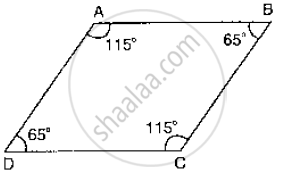# Which Pair of Lines in the Below Fig, is Parallel? Given Reasons. - Mathematics

Which pair of lines in the below fig, is parallel? Given reasons.#### Solution

∠A + ∠B =115 + 65 =180°

∴AB || BC          [As sum of co-interior angles we supplementary]

∠B + ∠C = 65 +115 = 180°

∴AB || CD         [As sum of interior angles are supplementary]

Concept: Parallel Lines and a Transversal
Is there an error in this question or solution?

#### APPEARS IN

RD Sharma Mathematics for Class 9
Chapter 10 Lines and Angles
Exercise 10.4 | Q 16 | Page 48